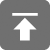# 工学1号馆

home

## HDOJ 1001 Sum Problem

Wu Yudong    May 07, 2018     HDOJ   542

Problem – 1001

 Problem Description Hey, welcome to HDOJ(Hangzhou Dianzi University Online Judge). In this problem, your task is to calculate SUM(n) = 1 + 2 + 3 + … + n. Input The input will consist of a series of integers n, one integer per line. Output For each case, output SUM(n) in one line, followed by a blank line. You may assume the result will be in the range of 32-bit signed integer. Sample Input 1 100 Sample Output 1 5050

#include<stdio.h>
#include<math.h>
#include<string.h>

int main()
{
long n;
while(scanf("%ld",&n)!=EOF)
printf("%ld\n\n", n%2? (n+1)/2*n: n/2*(n+1));
return 0;
}